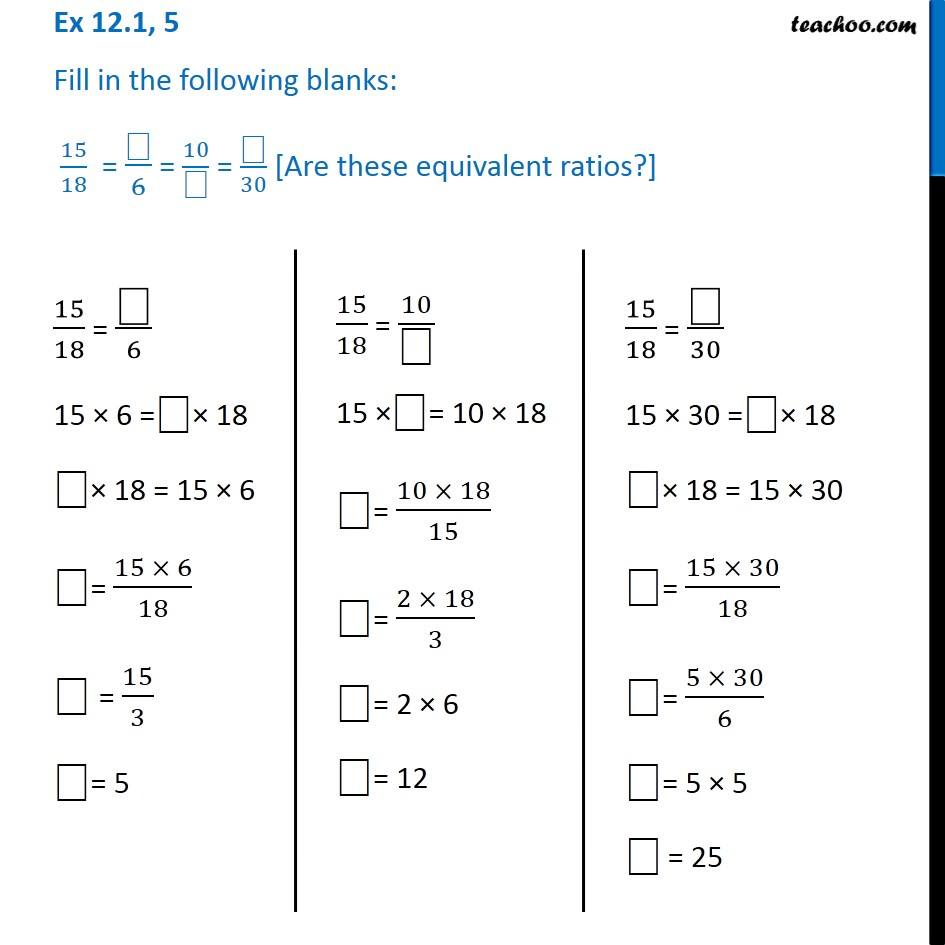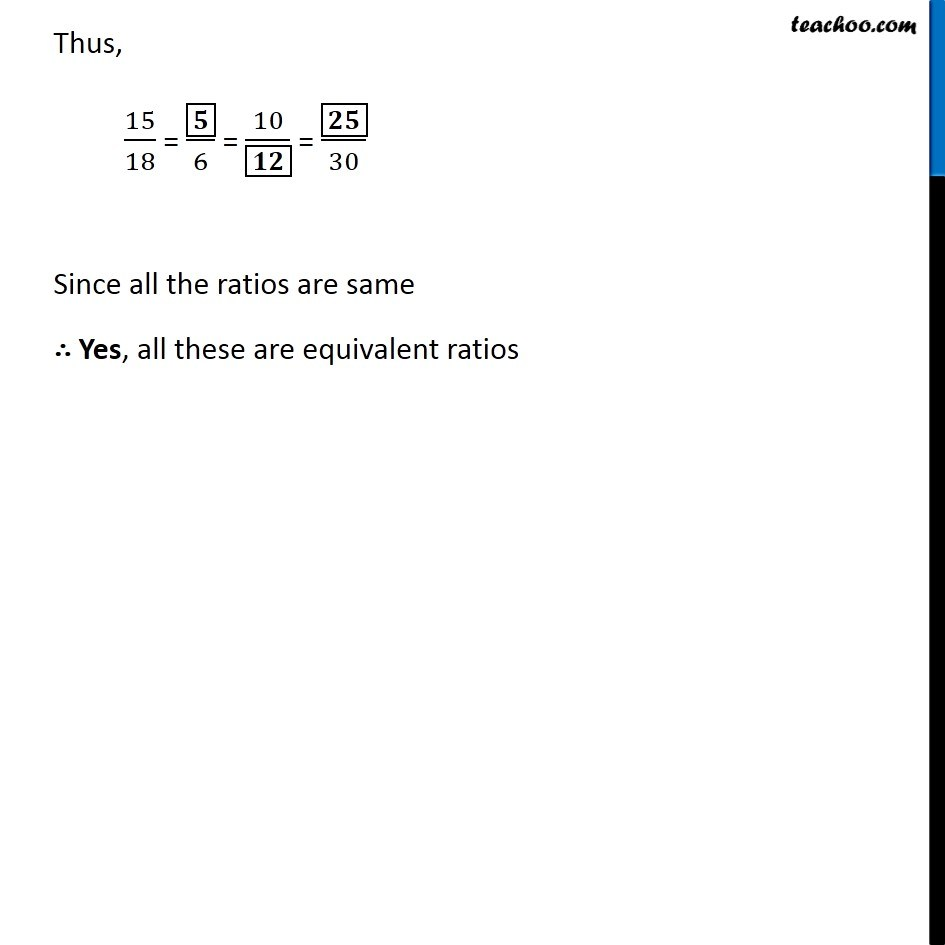1. Chapter 12 Class 6 Ratio And Proportion
2. Concept wise
3. Equivalent ratios

Transcript

Ex 12.1, 5 Fill in the following blanks: 15/18 = ⎕/6 = 10/⎕ = ⎕/30 [Are these equivalent ratios?] 15/18 = "⎕" /6 15 × 6 ="⎕"× 18 "⎕"× 18 = 15 × 6 "⎕"= (15 × 6)/18 "⎕" = 15/3 "⎕"= 5 15/18 = 10/"⎕" 15 ×"⎕"= 10 × 18 "⎕"= (10 × 18)/15 "⎕"= (2 × 18)/3 "⎕"= 2 × 6 "⎕"= 12 15/18 = "⎕" /30 15 × 30 ="⎕"× 18 "⎕"× 18 = 15 × 30 "⎕"= (15 × 30)/18 "⎕"= (5 × 30)/6 "⎕"= 5 × 5 "⎕" = 25 Thus, 15/18 = ▭𝟓/6 = 10/▭𝟏𝟐 = ▭𝟐𝟓/30 Since all the ratios are same ∴ Yes, all these are equivalent ratios

Equivalent ratios